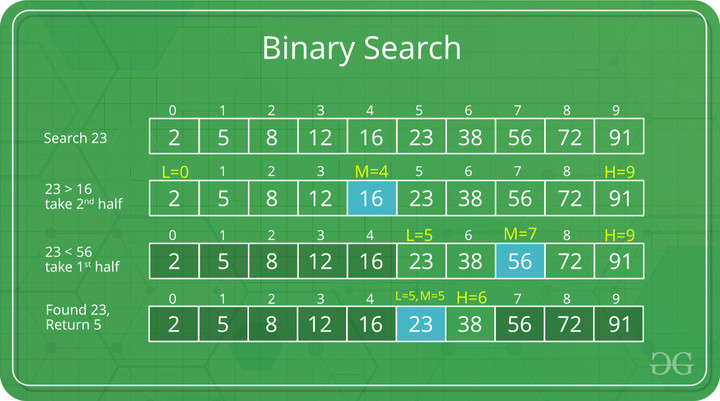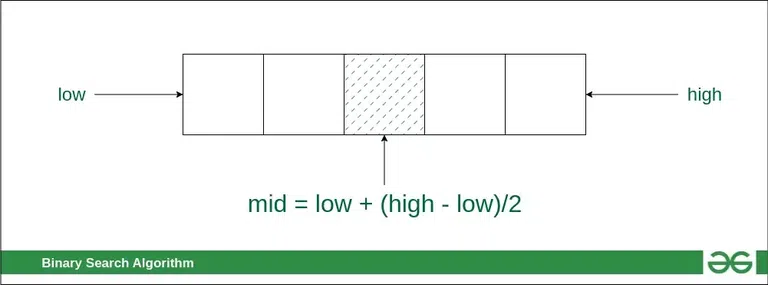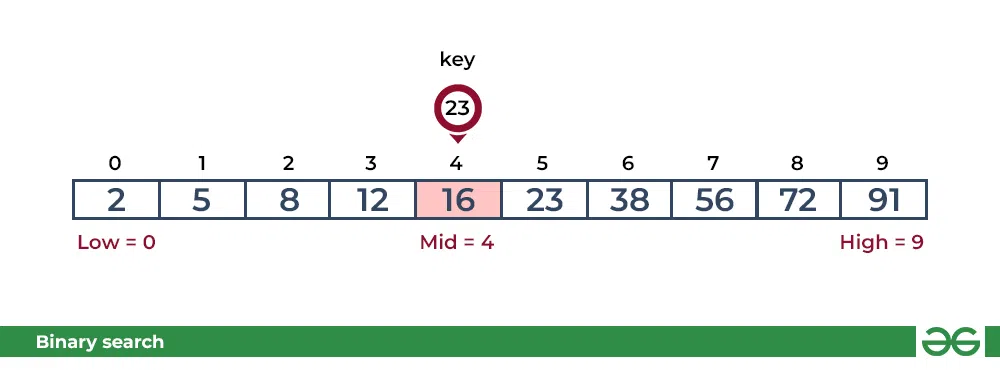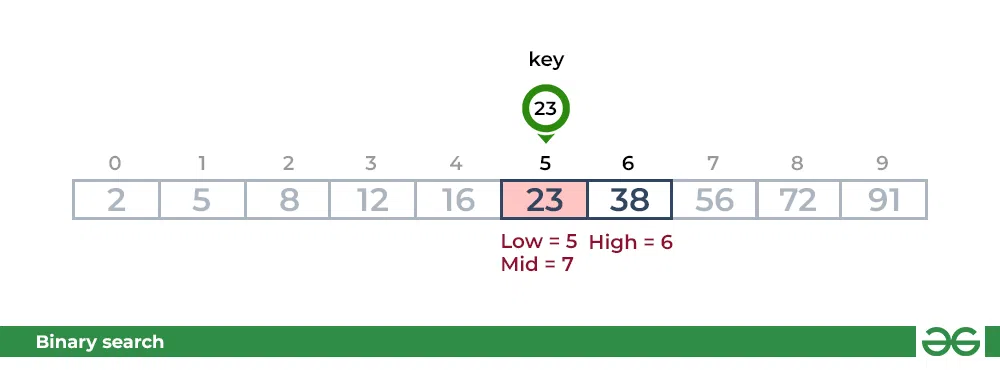GeeksforGeeks App
Open AppBrowser
Continue

# Binary Search – Data Structure and Algorithm Tutorials

Binary Search is defined as a searching algorithm used in a sorted array by repeatedly dividing the search interval in half. The idea of binary search is to use the information that the array is sorted and reduce the time complexity to O(log N).Example of Binary Search Algorithm

## Conditions for when to apply Binary Search in a Data Structure:

To apply Binary Search algorithm:

• The data structure must be sorted.
• Access to any element of the data structure takes constant time.

## Binary Search Algorithm:

In this algorithm,Finding the middle index “mid” in Binary Search Algorithm

• Compare the middle element of the search space with the key.
• If the key is found at middle element, the process is terminated.
• If the key is not found at middle element, choose which half will be used as the next search space.
• If the key is smaller than the middle element, then the left side is used for next search.
• If the key is larger than the middle element, then the right side is used for next search.
• This process is continued until the key is found or the total search space is exhausted.

## How does Binary Search work?

To understand the working of binary search, consider the following illustration:

Consider an array arr[] = {2, 5, 8, 12, 16, 23, 38, 56, 72, 91}, and the target = 23.

First Step: Calculate the mid and compare the mid element with the key. If the key is less than mid element, move to left and if it is greater than the mid then move search space to the right.

• Key (i.e., 23) is greater than current mid element (i.e., 16). The search space moves to the right.Binary Search Algorithm : Compare key with 16

• Key is less than the current mid 56. The search space moves to the left.Binary Search Algorithm : Compare key with 56

Second Step: If the key matches the value of the mid element, the element is found and stop search.Binary Search Algorithm : Key matches with mid

Recommended Practice

## How to Implement Binary Search?

The Binary Search Algorithm can be implemented in the following two ways

• Iterative Binary Search Algorithm
• Recursive Binary Search Algorithm

Given below are the pseudocodes for the approaches.

## 1. Iterative  Binary Search Algorithm:

Here we use a while loop to continue the process of comparing the key and splitting the search space in two halves.

Implementation of Iterative  Binary Search Algorithm:

## C

 `// C program to implement iterative Binary Search``#include ` `// An iterative binary search function.``int` `binarySearch(``int` `arr[], ``int` `l, ``int` `r, ``int` `x)``{``    ``while` `(l <= r) {``        ``int` `m = l + (r - l) / 2;` `        ``// Check if x is present at mid``        ``if` `(arr[m] == x)``            ``return` `m;` `        ``// If x greater, ignore left half``        ``if` `(arr[m] < x)``            ``l = m + 1;` `        ``// If x is smaller, ignore right half``        ``else``            ``r = m - 1;``    ``}` `    ``// If we reach here, then element was not present``    ``return` `-1;``}` `// Driver code``int` `main(``void``)``{``    ``int` `arr[] = { 2, 3, 4, 10, 40 };``    ``int` `n = ``sizeof``(arr) / ``sizeof``(arr);``    ``int` `x = 10;``    ``int` `result = binarySearch(arr, 0, n - 1, x);``    ``(result == -1) ? ``printf``(``"Element is not present"``                            ``" in array"``)``                   ``: ``printf``(``"Element is present at "``                            ``"index %d"``,``                            ``result);``    ``return` `0;``}`

## C++

 `// C++ program to implement iterative Binary Search``#include ``using` `namespace` `std;` `// An iterative binary search function.``int` `binarySearch(``int` `arr[], ``int` `l, ``int` `r, ``int` `x)``{``    ``while` `(l <= r) {``        ``int` `m = l + (r - l) / 2;` `        ``// Check if x is present at mid``        ``if` `(arr[m] == x)``            ``return` `m;` `        ``// If x greater, ignore left half``        ``if` `(arr[m] < x)``            ``l = m + 1;` `        ``// If x is smaller, ignore right half``        ``else``            ``r = m - 1;``    ``}` `    ``// If we reach here, then element was not present``    ``return` `-1;``}` `// Driver code``int` `main(``void``)``{``    ``int` `arr[] = { 2, 3, 4, 10, 40 };``    ``int` `x = 10;``    ``int` `n = ``sizeof``(arr) / ``sizeof``(arr);``    ``int` `result = binarySearch(arr, 0, n - 1, x);``    ``(result == -1)``        ``? cout << ``"Element is not present in array"``        ``: cout << ``"Element is present at index "` `<< result;``    ``return` `0;``}`

## Java

 `// Java implementation of iterative Binary Search` `import` `java.io.*;` `class` `BinarySearch {``  ` `    ``// Returns index of x if it is present in arr[].``    ``int` `binarySearch(``int` `arr[], ``int` `x)``    ``{``        ``int` `l = ``0``, r = arr.length - ``1``;``        ``while` `(l <= r) {``            ``int` `m = l + (r - l) / ``2``;` `            ``// Check if x is present at mid``            ``if` `(arr[m] == x)``                ``return` `m;` `            ``// If x greater, ignore left half``            ``if` `(arr[m] < x)``                ``l = m + ``1``;` `            ``// If x is smaller, ignore right half``            ``else``                ``r = m - ``1``;``        ``}` `        ``// If we reach here, then element was``        ``// not present``        ``return` `-``1``;``    ``}` `    ``// Driver code``    ``public` `static` `void` `main(String args[])``    ``{``        ``BinarySearch ob = ``new` `BinarySearch();``        ``int` `arr[] = { ``2``, ``3``, ``4``, ``10``, ``40` `};``        ``int` `n = arr.length;``        ``int` `x = ``10``;``        ``int` `result = ob.binarySearch(arr, x);``        ``if` `(result == -``1``)``            ``System.out.println(``                ``"Element is not present in array"``);``        ``else``            ``System.out.println(``"Element is present at "``                               ``+ ``"index "` `+ result);``    ``}``}`

## Python3

 `# Python3 code to implement iterative Binary``# Search.`  `# It returns location of x in given array arr``def` `binarySearch(arr, l, r, x):` `    ``while` `l <``=` `r:` `        ``mid ``=` `l ``+` `(r ``-` `l) ``/``/` `2` `        ``# Check if x is present at mid``        ``if` `arr[mid] ``=``=` `x:``            ``return` `mid` `        ``# If x is greater, ignore left half``        ``elif` `arr[mid] < x:``            ``l ``=` `mid ``+` `1` `        ``# If x is smaller, ignore right half``        ``else``:``            ``r ``=` `mid ``-` `1` `    ``# If we reach here, then the element``    ``# was not present``    ``return` `-``1`  `# Driver Code``if` `__name__ ``=``=` `'__main__'``:``    ``arr ``=` `[``2``, ``3``, ``4``, ``10``, ``40``]``    ``x ``=` `10` `    ``# Function call``    ``result ``=` `binarySearch(arr, ``0``, ``len``(arr)``-``1``, x)``    ``if` `result !``=` `-``1``:``        ``print``(``"Element is present at index"``, result)``    ``else``:``        ``print``(``"Element is not present in array"``)`

## C#

 `// C# implementation of iterative Binary Search``using` `System;` `class` `GFG {``    ` `    ``// Returns index of x if it is present in arr[]``    ``static` `int` `binarySearch(``int``[] arr, ``int` `x)``    ``{``        ``int` `l = 0, r = arr.Length - 1;``        ``while` `(l <= r) {``            ``int` `m = l + (r - l) / 2;` `            ``// Check if x is present at mid``            ``if` `(arr[m] == x)``                ``return` `m;` `            ``// If x greater, ignore left half``            ``if` `(arr[m] < x)``                ``l = m + 1;` `            ``// If x is smaller, ignore right half``            ``else``                ``r = m - 1;``        ``}` `        ``// If we reach here, then element was``        ``// not present``        ``return` `-1;``    ``}` `    ``// Driver code``    ``public` `static` `void` `Main()``    ``{``        ``int``[] arr = { 2, 3, 4, 10, 40 };``        ``int` `n = arr.Length;``        ``int` `x = 10;``        ``int` `result = binarySearch(arr, x);``        ``if` `(result == -1)``            ``Console.WriteLine(``                ``"Element is not present in array"``);``        ``else``            ``Console.WriteLine(``"Element is present at "``                              ``+ ``"index "` `+ result);``    ``}``}`

## PHP

 ``

## Javascript

 `// Program to implement iterative Binary Search` ` ` `// A iterative binary search function. It returns``// location of x in given array arr[l..r] is present,``// otherwise -1` ` ``function` `binarySearch(arr, x)``{   ``    ``let l = 0;``    ``let r = arr.length - 1;``    ``let mid;``    ``while` `(r >= l) {``         ``mid = l + Math.floor((r - l) / 2);`` ` `        ``// If the element is present at the middle``        ``// itself``        ``if` `(arr[mid] == x)``            ``return` `mid;`` ` `        ``// If element is smaller than mid, then``        ``// it can only be present in left subarray``        ``if` `(arr[mid] > x)``            ``r = mid - 1;``            ` `        ``// Else the element can only be present``        ``// in right subarray``        ``else``            ``l = mid + 1;``    ``}`` ` `    ``// We reach here when element is not``    ``// present in array``    ``return` `-1;``}` `    ``arr =``new` `Array(2, 3, 4, 10, 40);``    ``x = 10;``    ``n = arr.length;``    ``result = binarySearch(arr, x);``    ` `(result == -1) ? console.log(``"Element is not present in array"``)``               ``: console.log (``"Element is present at index "` `+ result);``               ` `// This code is contributed by simranarora5sos and rshuklabbb`

Output

`Element is present at index 3`

Time Complexity: O(log N)
Auxiliary Space: O(1)

## 2. Recursive  Binary Search Algorithm:

Create a recursive function and compare the mid of the search space with the key. And based on the result either return the index where the key is found or call the recursive function for the next search space.

Implementation of Recursive  Binary Search Algorithm:

## C

 `// C program to implement recursive Binary Search``#include ` `// A recursive binary search function. It returns``// location of x in given array arr[l..r] is present,``// otherwise -1``int` `binarySearch(``int` `arr[], ``int` `l, ``int` `r, ``int` `x)``{``    ``if` `(r >= l) {``        ``int` `mid = l + (r - l) / 2;` `        ``// If the element is present at the middle``        ``// itself``        ``if` `(arr[mid] == x)``            ``return` `mid;` `        ``// If element is smaller than mid, then``        ``// it can only be present in left subarray``        ``if` `(arr[mid] > x)``            ``return` `binarySearch(arr, l, mid - 1, x);` `        ``// Else the element can only be present``        ``// in right subarray``        ``return` `binarySearch(arr, mid + 1, r, x);``    ``}` `    ``// We reach here when element is not``    ``// present in array``    ``return` `-1;``}` `// Driver code``int` `main()``{``    ``int` `arr[] = { 2, 3, 4, 10, 40 };``    ``int` `n = ``sizeof``(arr) / ``sizeof``(arr);``    ``int` `x = 10;``    ``int` `result = binarySearch(arr, 0, n - 1, x);``    ``(result == -1)``        ``? ``printf``(``"Element is not present in array"``)``        ``: ``printf``(``"Element is present at index %d"``, result);``    ``return` `0;``}`

## C++

 `// C++ program to implement recursive Binary Search``#include ``using` `namespace` `std;` `// A recursive binary search function. It returns``// location of x in given array arr[l..r] is present,``// otherwise -1``int` `binarySearch(``int` `arr[], ``int` `l, ``int` `r, ``int` `x)``{``    ``if` `(r >= l) {``        ``int` `mid = l + (r - l) / 2;` `        ``// If the element is present at the middle``        ``// itself``        ``if` `(arr[mid] == x)``            ``return` `mid;` `        ``// If element is smaller than mid, then``        ``// it can only be present in left subarray``        ``if` `(arr[mid] > x)``            ``return` `binarySearch(arr, l, mid - 1, x);` `        ``// Else the element can only be present``        ``// in right subarray``        ``return` `binarySearch(arr, mid + 1, r, x);``    ``}` `    ``// We reach here when element is not``    ``// present in array``    ``return` `-1;``}` `// Driver code``int` `main()``{``    ``int` `arr[] = { 2, 3, 4, 10, 40 };``    ``int` `x = 10;``    ``int` `n = ``sizeof``(arr) / ``sizeof``(arr);``    ``int` `result = binarySearch(arr, 0, n - 1, x);``    ``(result == -1)``        ``? cout << ``"Element is not present in array"``        ``: cout << ``"Element is present at index "` `<< result;``    ``return` `0;``}`

## Java

 `// Java implementation of recursive Binary Search``class` `BinarySearch {` `    ``// Returns index of x if it is present in arr[l..``    ``// r], else return -1``    ``int` `binarySearch(``int` `arr[], ``int` `l, ``int` `r, ``int` `x)``    ``{``        ``if` `(r >= l) {``            ``int` `mid = l + (r - l) / ``2``;` `            ``// If the element is present at the``            ``// middle itself``            ``if` `(arr[mid] == x)``                ``return` `mid;` `            ``// If element is smaller than mid, then``            ``// it can only be present in left subarray``            ``if` `(arr[mid] > x)``                ``return` `binarySearch(arr, l, mid - ``1``, x);` `            ``// Else the element can only be present``            ``// in right subarray``            ``return` `binarySearch(arr, mid + ``1``, r, x);``        ``}` `        ``// We reach here when element is not present``        ``// in array``        ``return` `-``1``;``    ``}` `    ``// Driver code``    ``public` `static` `void` `main(String args[])``    ``{``        ``BinarySearch ob = ``new` `BinarySearch();``        ``int` `arr[] = { ``2``, ``3``, ``4``, ``10``, ``40` `};``        ``int` `n = arr.length;``        ``int` `x = ``10``;``        ``int` `result = ob.binarySearch(arr, ``0``, n - ``1``, x);``        ``if` `(result == -``1``)``            ``System.out.println(``                ``"Element is not present in array"``);``        ``else``            ``System.out.println(``                ``"Element is present at index "` `+ result);``    ``}``}``/* This code is contributed by Rajat Mishra */`

## Python3

 `# Python3 Program for recursive binary search.`  `# Returns index of x in arr if present, else -1``def` `binarySearch(arr, l, r, x):` `    ``# Check base case``    ``if` `r >``=` `l:` `        ``mid ``=` `l ``+` `(r ``-` `l) ``/``/` `2` `        ``# If element is present at the middle itself``        ``if` `arr[mid] ``=``=` `x:``            ``return` `mid` `        ``# If element is smaller than mid, then it``        ``# can only be present in left subarray``        ``elif` `arr[mid] > x:``            ``return` `binarySearch(arr, l, mid``-``1``, x)` `        ``# Else the element can only be present``        ``# in right subarray``        ``else``:``            ``return` `binarySearch(arr, mid ``+` `1``, r, x)` `    ``# Element is not present in the array``    ``else``:``        ``return` `-``1`  `# Driver Code``if` `__name__ ``=``=` `'__main__'``:``    ``arr ``=` `[``2``, ``3``, ``4``, ``10``, ``40``]``    ``x ``=` `10``    ` `    ``# Function call``    ``result ``=` `binarySearch(arr, ``0``, ``len``(arr)``-``1``, x)``    ` `    ``if` `result !``=` `-``1``:``        ``print``(``"Element is present at index"``, result)``    ``else``:``        ``print``(``"Element is not present in array"``)`

## C#

 `// C# implementation of recursive Binary Search``using` `System;` `class` `GFG {` `    ``// Returns index of x if it is present in``    ``// arr[l..r], else return -1``    ``static` `int` `binarySearch(``int``[] arr, ``int` `l, ``int` `r, ``int` `x)``    ``{``        ``if` `(r >= l) {``            ``int` `mid = l + (r - l) / 2;` `            ``// If the element is present at the``            ``// middle itself``            ``if` `(arr[mid] == x)``                ``return` `mid;` `            ``// If element is smaller than mid, then``            ``// it can only be present in left subarray``            ``if` `(arr[mid] > x)``                ``return` `binarySearch(arr, l, mid - 1, x);` `            ``// Else the element can only be present``            ``// in right subarray``            ``return` `binarySearch(arr, mid + 1, r, x);``        ``}` `        ``// We reach here when element is not present``        ``// in array``        ``return` `-1;``    ``}` `    ``// Driver code``    ``public` `static` `void` `Main()``    ``{` `        ``int``[] arr = { 2, 3, 4, 10, 40 };``        ``int` `n = arr.Length;``        ``int` `x = 10;` `        ``int` `result = binarySearch(arr, 0, n - 1, x);` `        ``if` `(result == -1)``            ``Console.WriteLine(``                ``"Element is not present in arrau"``);``        ``else``            ``Console.WriteLine(``"Element is present at index "``                              ``+ result);``    ``}``}` `// This code is contributed by Sam007.`

## PHP

 `= ``\$l``)``{``        ``\$mid` `= ``ceil``(``\$l` `+ (``\$r` `- ``\$l``) / 2);` `        ``// If the element is present``        ``// at the middle itself``        ``if` `(``\$arr``[``\$mid``] == ``\$x``)``            ``return` `floor``(``\$mid``);` `        ``// If element is smaller than``        ``// mid, then it can only be``        ``// present in left subarray``        ``if` `(``\$arr``[``\$mid``] > ``\$x``)``            ``return` `binarySearch(``\$arr``, ``\$l``,``                                ``\$mid` `- 1, ``\$x``);` `        ``// Else the element can only``        ``// be present in right subarray``        ``return` `binarySearch(``\$arr``, ``\$mid` `+ 1,``                            ``\$r``, ``\$x``);``}` `// We reach here when element``// is not present in array``return` `-1;``}` `// Driver Code``\$arr` `= ``array``(2, 3, 4, 10, 40);``\$n` `= ``count``(``\$arr``);``\$x` `= 10;``\$result` `= binarySearch(``\$arr``, 0, ``\$n` `- 1, ``\$x``);``if``((``\$result` `== -1))``echo` `"Element is not present in array"``;``else``echo` `"Element is present at index "``,``                            ``\$result``;``                            ` `// This code is contributed by anuj_67.``?>`

## Javascript

 `// JavaScript program to implement recursive Binary Search` `// A recursive binary search function. It returns``// location of x in given array arr[l..r] is present,``// otherwise -1``function` `binarySearch(arr, l, r, x){``    ``if` `(r >= l) {``        ``let mid = l + Math.floor((r - l) / 2);` `        ``// If the element is present at the middle``        ``// itself``        ``if` `(arr[mid] == x)``            ``return` `mid;` `        ``// If element is smaller than mid, then``        ``// it can only be present in left subarray``        ``if` `(arr[mid] > x)``            ``return` `binarySearch(arr, l, mid - 1, x);` `        ``// Else the element can only be present``        ``// in right subarray``        ``return` `binarySearch(arr, mid + 1, r, x);``    ``}` `    ``// We reach here when element is not``    ``// present in array``    ``return` `-1;``}` `let arr = [ 2, 3, 4, 10, 40 ];``let x = 10;``let n = arr.length``let result = binarySearch(arr, 0, n - 1, x);``(result == -1) ? console.log( ``"Element is not present in array"``)``               ``: console.log(``"Element is present at index "` `+result);`

Output

`Element is present at index 3`

## Complexity Analysis of Binary Search:

• Time Complexity:
• Best Case: O(1)
• Average Case: O(log N)
• Worst Case: O(log N)
• Auxiliary Space: O(1), If the recursive call stack is considered then the auxiliary space will be O(logN).

• Binary search is faster than linear search, especially for large arrays.
• More efficient than other searching algorithms with a similar time complexity, such as interpolation search or exponential search.
• Binary search is well-suited for searching large datasets that are stored in external memory, such as on a hard drive or in the cloud.

## Drawbacks of Binary Search:

• The array should be sorted.
• Binary search requires that the data structure being searched be stored in contiguous memory locations.
• Binary search requires that the elements of the array be comparable, meaning that they must be able to be ordered.

## Applications of Binary Search:

• Binary search can be used as a building block for more complex algorithms used in machine learning, such as algorithms for training neural networks or finding the optimal hyperparameters for a model.
• It can be used for searching in computer graphics such as algorithms for ray tracing or texture mapping.
• It can be used for searching a database.

My Personal Notes arrow_drop_up